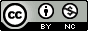## Monday, July 27, 2009

### R Snippet for Sampling from a Dataframe

It took me a while to figure this out, so I thought I'd share.

I have a dataframe with millions of observations in it, and I want to estimate a density distribution, which is a memory intensive process. Running my kde2d function on the full dataframe throws and error -- R tries to allocate a vector that is gigabytes in size. A reasonable alternative is to run the function on a smaller subset of the data. R has a nifty sample function, but it is designed to randomly sample from a vector, not a 2D dataframe. The sample function CAN work for this though, like so:
sub <- Dataset[sample(1:dim(Dataset), size=100000, replace=FALSE),]

Now, sub contains a subset from my 2D dataframe containing 100000 observations. This example was a sample without replacement, but if you set replace=TRUE, you can get a sample with replacement also.

#### 8 comments:

1.nice! or (taking the defaults for sample and wanting to sample at 10%):

sub=Dataset[sample(nrow(Dataset)*0.10),]

2.Cheers for the handy tip. This saved me some time.

3.Thank you for sharing!

4.Thank you for sharing. If there is a weight associated with each observation in the data frame, how can I sample from this data frame? Thanks.

1.A weight in the sense that you want this sample to be drawn proportional to its weight? If that's the case the simplest solution I can think of is to create a new data frame with the sample repeated proportional to its weight and use the code above. Simple but inefficient (could be problematic for very large data). If you need efficiency without creating a new data frame you might try drawing a uniform random number, comparing it to the weight, and selecting the sample if it is above the weight, or something like that.

5.Thanks for sharing this, this is great. What if I wanted to subset in a way that there was no duplication between subsets, making 3 for training/validation/holdout? The 3 subsets should add up to the original dataset. I can make a vector of T/F based on < Year, but this masks, or captures while masking (if that makes any sense), any time trend. So, I want to randomly sample, but in a way that there won't be any duplication between subsets.

Thanks!

1.Actually, I found one of your other posts, which referred me to the caret package, which does everything I could want and so much more. Thanks though...many great references on this site.

2.Yes, the caret package is great. Glad you found something that will work for you.

Note: Only a member of this blog may post a comment.Getting Genetics Done by Stephen Turner is licensed under a Creative Commons Attribution-NonCommercial 3.0 Unported License.